• python有趣的图案代码
千次阅读
2020-12-05 09:49:35

Python 练习实例65

题目：一个最优美的图案。

程序分析：无。

程序源代码：#!/usr/bin/python

# -*- coding: UTF-8 -*-

import math

class PTS:

def __init__(self):

self.x = 0

self.y = 0

points = []

def LineToDemo():

from Tkinter import *

screenx = 400

screeny = 400

canvas = Canvas(width = screenx,height = screeny,bg = 'white')

AspectRatio = 0.85

MAXPTS = 15

h = screeny

w = screenx

xcenter = w / 2

ycenter = h / 2

radius = (h - 30) / (AspectRatio * 2) - 20

step = 360 / MAXPTS

angle = 0.0

for i in range(MAXPTS):

rads = angle * math.pi / 180.0

p = PTS()

angle += step

points.append(p)

for i in range(MAXPTS):

for j in range(i,MAXPTS):

canvas.create_line(points[i].x,points[i].y,points[j].x,points[j].y)

canvas.pack()

mainloop()

if __name__ == '__main__':

LineToDemo()

以上实例输出结果为：更多相关内容
• python简单实现一个图形的绘制，可以自己设置图形的大小和尺寸
• python画生日蛋糕，并可自行设置生日祝福对象及祝福语。
• 汉诺塔是传统的智力游戏，与华容道、魔方等类似。这是汉诺塔游戏的Python代码，使用了基本的递归方式实现汉诺塔求解问题。 欢迎大家下载。
• Python实现连连看游戏，有趣，形象，可以给初学者很多启发，能够学到很多东西！Python实现小游戏实例
• 目录一、离散型变量的可视化1 饼图1.1 matplotlib模块1.2 panda模块2 条形图2.1 matplotlib模块2.1.1 垂直或水平条形图2.1.2 堆叠条形图2.1.3 水平交错条形图2.2 pandas模块2.2.1 垂直条形图2.2.2 水平交错条形图2.3...
• 下面分享25个有趣且实用的Python单行代码，欢迎大家点赞、收藏，支持！ 1. 交换两个变量 a = 4 b = 5 a,b = b,a ## print(a,b) #结果：5 4 2. 多变量赋值 a,b,c = 4,5.5,'Hello' ## print(a,b,c) #结果： 4...

在用Python的第一天，便对它的简单性、流行性及其著名的单行代码着迷。

下面分享25个有趣且实用的Python单行代码，欢迎大家点赞、收藏，支持！

## 1. 交换两个变量

a = 4
b = 5
a,b = b,a ##
print(a,b)
#结果：5 4

## 2. 多变量赋值

a,b,c = 4,5.5,'Hello' ##
print(a,b,c) #结果： 4 5.5 hello

增加一点点难度：

a,b,*c = [1,2,3,4,5]  ##
print(a,b,c) #结果： 1 2 [3,4,5]

## 3. 列表偶数求和

a = [1,2,3,4,5,6]
s = sum([num for num in a if num%2 == 0]) ##
print(s) #结果： 12

## 4. 列表删除多元素

del 是 Python 中用于从列表中删除值的关键字。

a = [1,2,3,4,5]
del a[1::2] ##
print(a) #结果：[1, 3, 5]

此处需要理解L[start:end:step]: - start为开始位置，省略时为0。 - end为结束位置，省略时为列表长度； - step步长，省略时为1，如果有负号- 表示按列表自右到左的方向迭代。

## 5. 读取文件

最常用的方式

with open("data.txt") as f: ##
lst=[line.strip() for line in f]
print(lst)

更简单、更短的方法

lst = list(open('data.txt')) ##
print(lst)

也可以

lst = [line.strip() for line in open('data.txt')] ##
print(lst)

## 6. 将数据写入文件

with open("data.txt",'a') as f:
f.write("Python is awsome")

## 7. 创建列表

range()函数可以用于生成一系列整数:

lst = [i for i in range(0,10)] ##
print(lst)

单行列表推导，快速创建:

lst = [("Hello "+i) for i in ['Karl','Abhay','Zen']] ##
print(lst)

## 8. 映射列表

有时需要改变一个列表中所有元素的数据类型。比较直观的想法是使用循环：先访问列表中的所有元素，然后逐个更改元素的数据类型。这种方法中规中矩，但巧用 map一一映射，耳目一新。

list(map(int,['1','2','3'])) ##
list(map(float,[1,2,3])) ##
[float(i) for i in [1,2,3]] ##   

## 9. 快速创建集合

我们可用创建列表的方法快速创建集合:

#### 范围内所有偶数的平方
s = {x**2 for x in range(10) if x%2==0} ##
print(s) #结果：{0, 4, 16, 36, 64}

## 10. FizzBuzz单行代码

有这样一个问题，需要编写一个程序来打印从 1 到 100 的数字。其中对于3的倍数，打印Fizz，对于 5 的倍数，打印Buzz

显而易见，可以使用循环和多个if-else 语句来实现。

如果使用java语言来完成，估计至少10行代码，但使用python，一行搞定！！！。

['FizzBu​​zz' if i%3==0 and i%5==0 else 'Fizz' if i%3==0 else 'Buzz' if i%5==0 else i for i in range(1,20 )] ##     

在上面的代码中，使用列表推导来运行一个从1 到 20 的循环，然后在循环的每次迭代中，检查数字： - 如果能同时被 3 和 5 整除，则用 FizzBu​​zz 替换; - 如果只能被 3 整除，我们用 Fizz 替换; - 如果只能被 5 整除，则用 Buzz 替换; - 否则仍然为原来的数字。

## 11. 回文数

回文是反转后看起来相同的数字或字符串。

text = 'level'
ispalindrome = text == text[::-1] ##
print(ispalindrome) ## True

## 12. 空格分隔整数到列表

input_string = '1 2 3 4 5'
lis = list(map(int,input_string.split())) ##
print(lis) ## [1, 2, 3, 4, 5]

## 13. Lambda 函数

lambda函数是匿名函数。一个 lambda 函数可以接受任意数量的参数，但只能是一个表达式。

下面是一个简单的lambda 函数，它接受一个数字，并返回数字的平方：

sqr = lambda x: x * x   ##
sqr(10) ## 100

## 14. 检查元素是否在列表中

num = 5
res = 'present' if num in [1,2,3,4,5] else 'not present' ##
print(res) ## present

## 15. 打印图案

用符号打印有趣的图案总是让我着迷。在python中，只需一行代码就可以绘制出惊人的图案:

n = 5
print('\n'.join(' ' * i for i in range(1, n + 1))) ##    

输出为:





## 16. 计算阶乘

import math
n = 6
print(math.factorial(n)) ## 720

## 17. 斐波那契数列

斐波那契数列，其中每个数字都是前面两个数字的总和。例如：最简单的斐波那契数列: 1, 1, 2, 3, 5, 8, 13。 我们可以使用列表推导创建一个范围内的斐波那契数列。

fibo = [0,1]
[fibo.append(fibo[-1]+fibo[-2]) for i in range(5)] ##
print(fibo) ## [0, 1, 1, 2, 3, 5, 8]

## 18. 生成素数

素数是一个只能被它自己和 1 整除的数。例如： 2,3,5,7。为了在一个范围内生成素数，可以使用带有过滤器filterlambda 的列表函数来生成素数。

prime = list(filter(lambda x:all(x % y != 0 for y in range(2, x)), range(2, 13))) ##
print(prime) ## [2, 3, 5, 7, 11, 13]

## 19. 最大数

findmax = lambda x,y: x if x > y else y ##
findmax(5,14) ## 14

## 20. 线性变换

有时需要将列表的元素缩放到 2倍或5倍。函数法实现：

def scale(lst, x):
return [i*x for i in lst] ##
scale([2,3,4], 2) ## [4, 6, 8]

## 21. 矩阵的转置

需要将所有行更改为列，反之亦然。在python中，可以使用zip函数在一行代码中转置矩阵。当然在numpy中，可以直接使用transpose函数。

a=[[1,2,3],
[4,5,6],
[7,8,9]]
transpose = [list(i) for i in zip(*a)] ##
print(transpose) ## [[1, 4, 7], [2, 5, 8], [3, 6, 9]]

## 22. 正则表达式--模式匹配

当需要知道文本中某个模式出现的次数时，在 python 中，有re库可以完成这些工作。

import re
res = re.findall('python','python is a programming language. python is python.')) ##
print(res) ## ['python', 'python', 'python']
当然 re库不仅仅上面这么简单。 re是一个非常有用的库，帮助我们更好地处理文本。

## 23. 文本替换

s = "python is a programming language.python is python"
s = s.replace("python",'Java') ##
print(s) ## "Java is a programming language.Java is Java"

## 24. 模拟抛硬币

当需要从给定的集合中中些随机进行选择，random.choice非常有用:

import random;
print(res) ## Head or Tail

## 25. 生成组合元组

groups = [(a, b) for a in ['a', 'b'] for b in [1, 2, 3]] ##
print(groups) ## [('a', 1), ('a', 2), ('a', 3), ('b', 1), ('b', 2), ('b', 3)]

## 小节

以上就是我们分享的25个有趣且实用的pyhton单行代码，希望充实你武器库！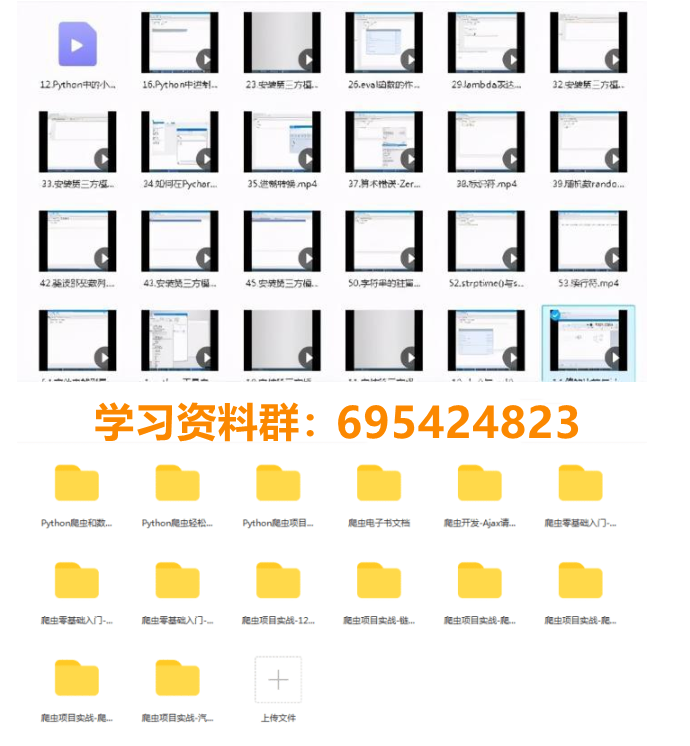展开全文• 主要是关于很常见的图形绘制的python代码，很适合python的初学者
• 是不是觉得非常挺炫酷的，但其实实现它的代码非常简单，仅仅10行Python代码就能实现。不过这都依赖于强大的turtle库。 3、多边形测试代码： import turtle #导入turtle库 t = turtle.Pen() #创建turtle对象 turtle....
• PS：部分图案参考了一些博客的一部分源代码。顺便证明一波我的审美T_T。。。没错，是时候证明一波了~~~Let's Go！相关文件密码: grsp开发工具Python版本：3.6.4相关模块：numpy模块；matplotlib模块；numba...

下载W3Cschool手机App，0基础随时随地学编程导语

花了点时间利用Python绘制了一些有意思的图案，也算是发掘了一下Python的可视化功能吧。。。

PS：

部分图案参考了一些博客的一部分源代码。

顺便证明一波我的审美T_T。。。

没错，是时候证明一波了~~~

Let's Go！

相关文件

密码: grsp

开发工具

Python版本：3.6.4

相关模块：

numpy模块；

matplotlib模块；

numba模块；

PIL模块；

以及一些Python自带的模块。

环境搭建

安装Python并添加到环境变量，pip安装需要的相关模块即可。

效果展示

原理没啥好说的，直接展示吧~~~

首先当然是一些逆天的数学分形图案啦~~~

（1）朱利亚分形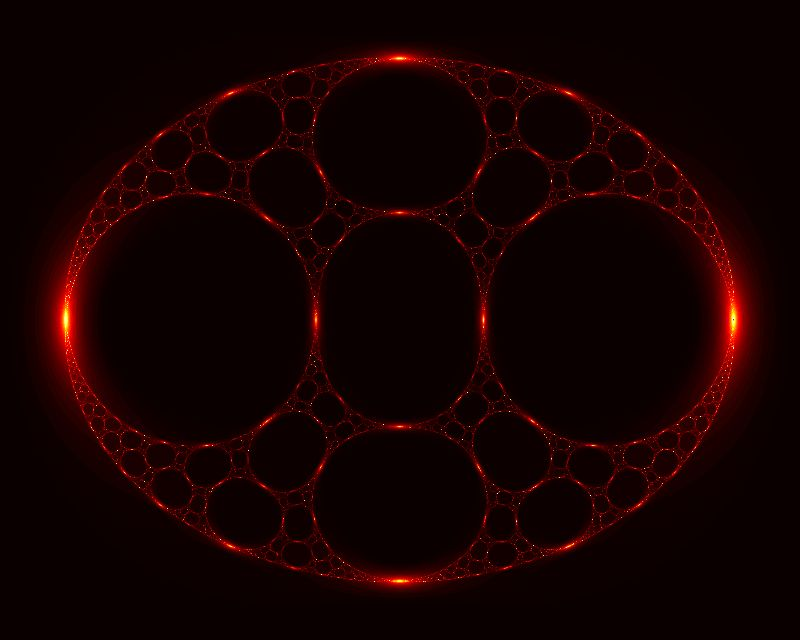（2）二十面体对称的万花筒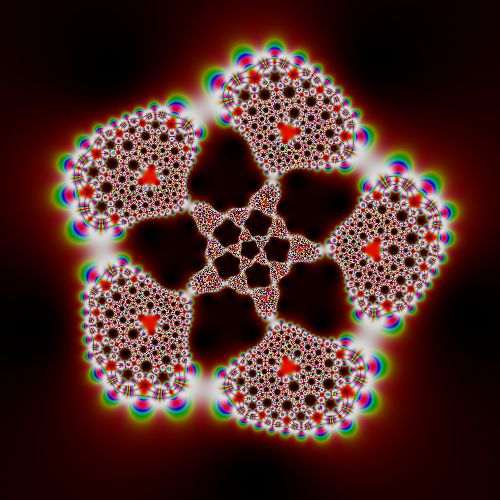（3）曼德勃罗集（4）牛顿分形其次，我也用Python的turtle模块画了一些有点意思的图案。

（1）漫天雪花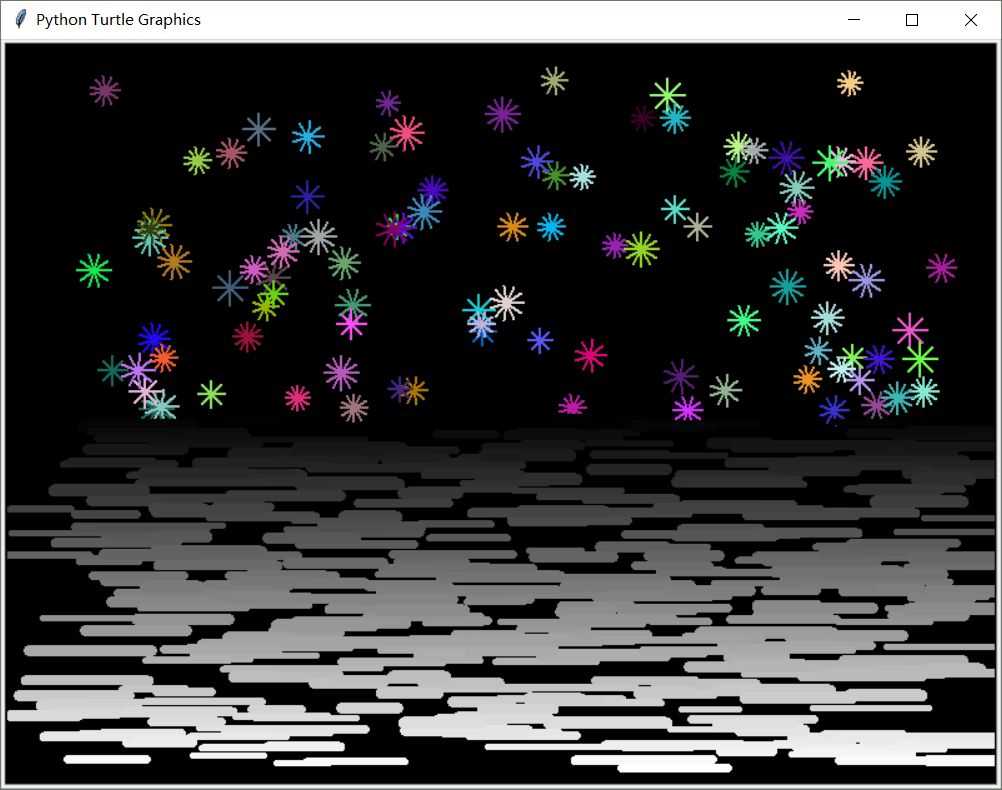（2）玫瑰

方案一：

方案二：

（3）爱心（T_T好吧有些非主流）

That's all...以后有机会再画其他的吧~~~

更多

Python的可视化库还是非常丰富的，功能也十分强大，有需要的朋友可以自己去学习一下。

譬如：

Matplotlib；

Pandas；

Bokeh；

Pygal等等。

展开全文• python一行代码实现打印打印心形图案python一行代码实现打印打印心形图案
• 使用python实现的图像金字塔获取程序，包括高斯金字塔和拉普拉斯金字塔，用于检验CNN输出的残差
• #getscreen().tracer(30, 0) #取消注释后，快速显示图案 heart(200, 0, 1) #画出第一颗心，前面两个参数控制心的位置，函数最后一个参数可控制心的大小 setheading(0) #使画笔的方向朝向x轴正方向 heart(-80, -...

1、绘制多彩圆圈

import turtle
colors = ["red","orange","yellow","green","blue","indigo","purple",'black']#颜色
for i in range(len(colors)):#循环画8个
c = colors[i]
turtle.color(c)#显示颜色
turtle.begin_fill()
turtle.circle(100)
turtle.rt(360/len(colors))#分割八次
turtle.end_fill()
turtle.done()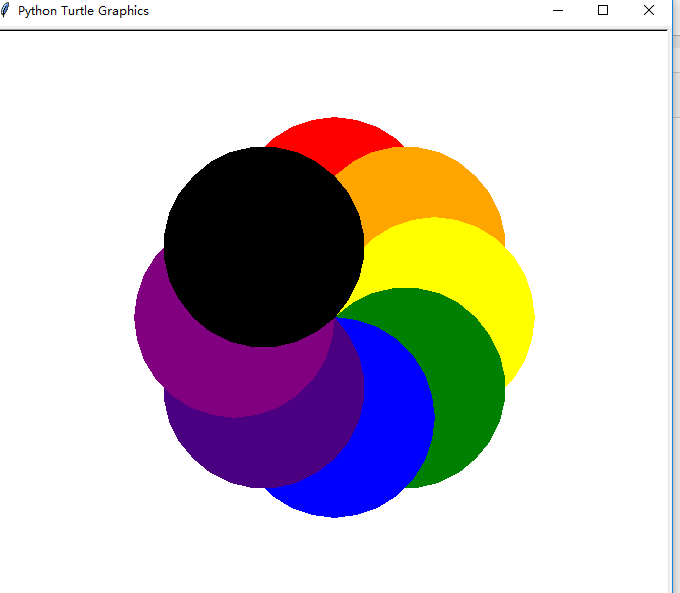2、画时钟

import turtle
from datetime import *

# 抬起画笔，向前运动一段距离放下
def Skip(step):
turtle.penup()
turtle.forward(step)
turtle.pendown()

def mkHand(name, length):
# 注册Turtle形状，建立表针Turtle
turtle.reset()
Skip(-length * 0.1)
# 开始记录多边形的顶点。当前的乌龟位置是多边形的第一个顶点。
turtle.begin_poly()
turtle.forward(length * 1.1)
# 停止记录多边形的顶点。当前的乌龟位置是多边形的最后一个顶点。将与第一个顶点相连。
turtle.end_poly()
# 返回最后记录的多边形。
handForm = turtle.get_poly()
turtle.register_shape(name, handForm)

def Init():
global secHand, minHand, hurHand, printer
# 重置Turtle指向北
turtle.mode("logo")
# 建立三个表针Turtle并初始化
mkHand("secHand", 135)
mkHand("minHand", 125)
mkHand("hurHand", 90)
secHand = turtle.Turtle()
secHand.shape("secHand")
minHand = turtle.Turtle()
minHand.shape("minHand")
hurHand = turtle.Turtle()
hurHand.shape("hurHand")

for hand in secHand, minHand, hurHand:
hand.shapesize(1, 1, 3)
hand.speed(0)

# 建立输出文字Turtle
printer = turtle.Turtle()
# 隐藏画笔的turtle形状
printer.hideturtle()
printer.penup()

# 建立表的外框
turtle.reset()
turtle.pensize(7)
for i in range(60):
if i % 5 == 0:
turtle.forward(20)

if i == 0:
turtle.write(int(12), align="center", font=("Courier", 14, "bold"))
elif i == 30:
Skip(25)
turtle.write(int(i/5), align="center", font=("Courier", 14, "bold"))
Skip(-25)
elif (i == 25 or i == 35):
Skip(20)
turtle.write(int(i/5), align="center", font=("Courier", 14, "bold"))
Skip(-20)
else:
turtle.write(int(i/5), align="center", font=("Courier", 14, "bold"))
else:
turtle.dot(5)
turtle.right(6)

def Week(t):
week = ["星期一", "星期二", "星期三",
"星期四", "星期五", "星期六", "星期日"]
return week[t.weekday()]

def Date(t):
y = t.year
m = t.month
d = t.day
return "%s %d%d" % (y, m, d)

def Tick():
# 绘制表针的动态显示
t = datetime.today()
second = t.second + t.microsecond * 0.000001
minute = t.minute + second / 60.0
hour = t.hour + minute / 60.0

turtle.tracer(False)
printer.forward(65)
printer.write(Week(t), align="center",
font=("Courier", 14, "bold"))
printer.back(130)
printer.write(Date(t), align="center",
font=("Courier", 14, "bold"))
printer.home()
turtle.tracer(True)

# 100ms后继续调用tick
turtle.ontimer(Tick, 100)

def main():
# 打开/关闭龟动画，并为更新图纸设置延迟。
turtle.tracer(False)
Init()
SetupClock(160)
turtle.tracer(True)
Tick()
turtle.mainloop()

if __name__ == "__main__":
main()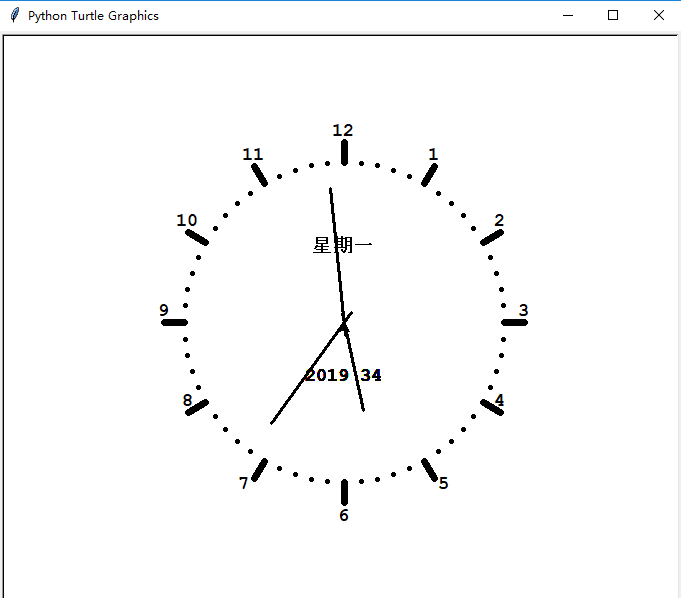from turtle import *
from time import sleep

def go_to(x, y):
up()
goto(x, y)
down()

def big_Circle(size):  #函数用于绘制心的大圆
speed(5)
for i in range(150):
forward(size)
right(0.3)

def small_Circle(size):  #函数用于绘制心的小圆
speed(10)
for i in range(210):
forward(size)
right(0.786)

def line(size):
speed(5)
forward(51*size)

def heart( x, y, size):
go_to(x, y)
left(150)
begin_fill()
line(size)
big_Circle(size)
small_Circle(size)
left(120)
small_Circle(size)
big_Circle(size)
line(size)
end_fill()

def arrow():
pensize(10)
go_to(-400, 0)
left(15)
forward(150)
go_to(339, 178)
forward(150)

pensize(1)
speed(5)
color('red', 'red')
begin_fill()
left(120)
forward(20)
right(150)
forward(35)
right(120)
forward(35)
right(150)
forward(20)
end_fill()

def main():
pensize(4)
color('red', 'pink')
#getscreen().tracer(30, 0) #取消注释后，快速显示图案
heart(200, 0, 1)          #画出第一颗心，前面两个参数控制心的位置，函数最后一个参数可控制心的大小
heart(-80, -100, 1.5)     #画出第二颗心
arrow()                   #画出穿过两颗心的直线
go_to(400, -300)
done()
main()展开全文• >>> print(" ".join(["".join([("Love"[(x-y)%4]if((x*0.05)**2+(y*0.1)**2-1)**3-(x*0.05)**2*(y*0.1)**3
• 本文实例为大家分享了Python生成树形图案的具体代码，供大家参考，具体内容如下 先看一下效果，见下图。 上面这颗大树是使用Python + Tkinter绘制的，主要原理为使用分形画树干、树枝，最终叶节点上画上绿色圆圈...
• 时间：2018-10-11概述：打印图形程序分析：... Python代码如下：#当然这样有些无耻print('''*************************''')#打印任意高度的菱形def diamond(h):if h%2==0:print('菱形的高度只能是奇数！')return#...
• 前言 Python 是一门简单易学且功能强大的编程...Python几段代码也能让我们做出几个有趣的动画 1、旋转动画 import turtle t = turtle.Pen() turtle.bgcolor("black") sides=6 colors=["red","yellow...编程语言
• 基于Python3.7实现，参考从入门到实践>。实现完整扫雷游戏逻辑，含随机雷分布，第一步必不踩雷，一片区域无雷自动点开，双键同时按下操作的逻辑，记分牌等等。 按下屏幕中间脸蛋重置游戏
• 本篇文章通过代码实例给大家详细分析了Python打印“菱形”星号代码方法，对此有需要的朋友参考下吧。
• ## Python画图案

千次阅读 2019-07-29 16:29:34
一.画五角星 import turtle t = turtle.Turtle() t.speed() #设置画笔速度最快 c=['red','green','purple','blue'] for x in range(5): t.color(c[x%4]) #设置颜色 t.forward(100) #画笔向前移动距离100 ...
• import matplotlib.pyplot as pltimport pandas as pdimport seaborn as snsimport numpy as np# 0、导入数据集df = pd.read_excel('first.xlsx', 'Sheet1')直方图fig = plt.figure()ax = fig.add_subplot(111)ax....
• 本文作者用简单明了的语言解释了三日k线的交易原则，也分享了如何用python绘制k线图的方法和代码。 关于日本k线交易据说日本人在十七世纪就已经运用技术分析的方法进行大米交易，一位名叫本间宗久的坂田大米贸易商...
• 使用python编写，实现将图片转换成相似的文字图案。生成的文本大小可以更改，程序定义四个参数：转换的图片地址 宽 高 生成的目标文件地址。...